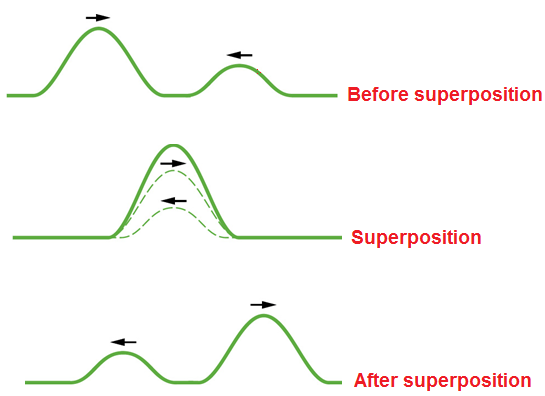# Principle of Superposition of Wave

When two or more than two waves travelling through a medium at the same time, the resultant displacement at any point is equal to the vector sum of their individual displacement caused by each wave separately at that point.i.e., y = y1 + y2 +…… yn

y = resultant

yn = individual displacement

If the displacement are along the same direction we can write,

y = y1 + y2+ y3 + ……yn

If two waves each of same amplitude undergo superposition, the result displacement is:

y = a + a = 2a (Constructive interference)

y = a – a = 0 (Destructive interference)

This principle is used to explain different wave phenomenon such as interference, beats etc as:

• When two waves having same frequency are moving in a same direction superpose, constructive interference is produced.
• When two waves in the opposite direction superpose, stationary waves are formed.
• When two waves of slightly different frequencies  are travelling in the same direction, beats are formed.

Do you like this article ? If yes then like otherwise dislike : 2